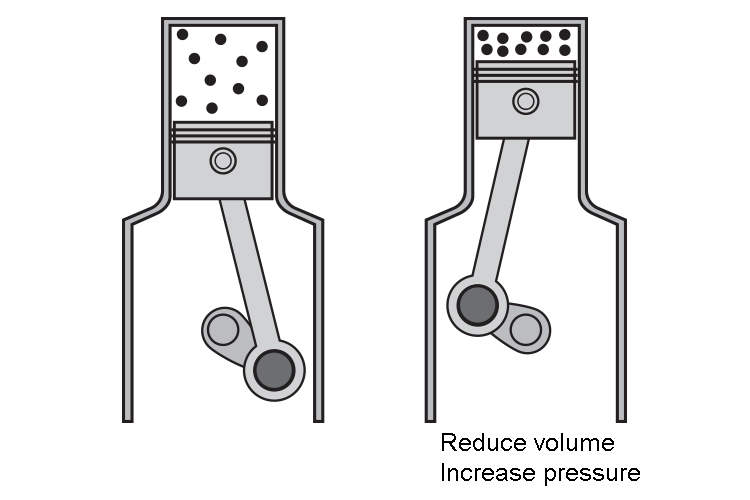# Boyle's law in use

We know that in Boyle's law

P_1\ \ V_1=P_2\ \ V_2

NOTE:

Temperature remains constant

Also when you sit on an exercise ball it will get smallerThis is Boyles law.

P_1 and V_1 refer to the pressure and volume before you sit on the exercise ball and P_2 and V_2 refer to the pressure and volume after you sit on the exercise ball.

Reducing the volume of a gas increases the pressure of the gas.Push the gas into a smaller volume and the gas particles will collide more frequently with the walls. They will exert more pressure.

NOTE 1:

The standard unit used to measure pressure is Pa (Pascals)

The standard unit used to measure volume is m^3 (metre cubed)

NOTE 2:

You can use other units for volume and pressure such as ml, pounds per square inch, mm of mercury (mm\ \Hg) or atm (atmospheres) as long as you use the same units either side of the equation.

Examples

1. A sample of gas is at atmospheric pressure (100,000 Pa) and has volume 50cm^3

Determine the new pressure if the volume is decreased to 35cm^3

A sample of gas is at atmospheric pressure (100,000 Pa) and has volume 50cm^3

Determine the new pressure if the volume is decreased to 35cm^3

PV=constant
P_1V_1=P_2V_2

Therefore  100,000xx50=P_2xx35

5,000,000=P_2xx35

Therefore  P_2=(5,000,000)/35

P_2=142,857\ \Pa

2. A sample of gas has a volume of 12.0\ \l at a pressure of 1.00  atm. If the pressure of gas is increased to 1000\ \mm\ \ Hg, what is the new volume of the gas?

sample of gas has a volume of 12.0\ \l at a pressure of 1.00 atm. If the pressure of gas is increased to 1000\ \mm\ \ Hg, what is the new volume of the gas?

P_1V_1=P_2V_2

But we must first get the two pressures in the same units of measurement

1\ \atm=760\ \mm\ \Hg

Therefore:

P_1=760
V_1=12
P_2=1000
V_2=?

760xx12=1000V_2

9120=1000V_2

9120/1000=V_2

V_2=9.12l

3. A carbon dioxide gas cylinder contains 180cm^3 of gas at a pressure of 6.3xx10^6 Pa. Calculate the volume of the gas at atmospheric pressure, 1.0xx10^5 Pa

A carbon dioxide gas cylinder contains 180cm^3 of gas at a pressure of 6.3xx10^6 Pa. Calculate the volume of the gas at atmospheric pressure, 1.0xx10^5 Pa

P_1V_1=P_2V_2

6.3xx10^6xx180=1.0xx10^5xxV_2

V_2=(6.3xx10^6xx180)/(1.0xx10^5)

Therefore V_2=11340\ \cm^3